# Test: Basic Concepts

## 20 Questions MCQ Test GATE ECE (Electronics) 2023 Mock Test Series | Test: Basic Concepts

Description
Attempt Test: Basic Concepts | 20 questions in 60 minutes | Mock test for Railways preparation | Free important questions MCQ to study GATE ECE (Electronics) 2023 Mock Test Series for Railways Exam | Download free PDF with solutions
QUESTION: 1

### If 120 C of charge passes through an electric conductor in 60 sec, the current in the conductor is:

Solution: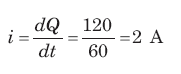QUESTION: 2

### A solid copper sphere, 10 cm in diameter is deprived of 1020 electrons by a charging scheme. The charge on the sphere is:

Solution:
• n = 1020
• Q = ne = e * 1020
= -16.02 C
• Charge on sphere will be positive as this electric charge is removed.
QUESTION: 3

### A lightning bolt carrying 15,000 A lasts for 100 μs. If the lightning strikes an airplane flying at 2 km, the charge deposited on the plane is:

Solution:

ΔQ = i x Δ t = 15000 x 100μ = 1.5 C

QUESTION: 4

The energy required to move 120 coulombs through 3 V is:

Solution:
• W = QV
• W = 120 * 3 = 360 J
QUESTION: 5

Find the value of i.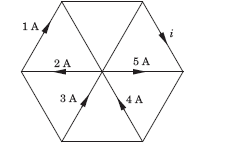Solution: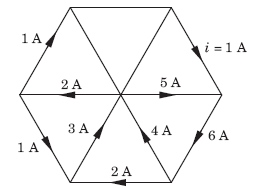• Based on kirchoffs current law, we know that incoming currents at a node is equals to outgoing currents.
• So, based on this 4 and 3 will get canceled with 5 and 2.
• So, finally i = 1.
QUESTION: 6

In the circuit given, a charge of 600 C is delivered to the 100 V source in a 1 minute. The value of v1 must be: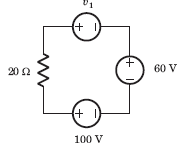Solution:
• In order for 600 C charge to be delivered to the 100 V source, the current must be anticlockwise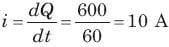• Applying KVL we get:
v1 + 60 - 100 = 10 x 20
v1 = 240 V
QUESTION: 7

In the circuit of the fig the value of the voltage source E is: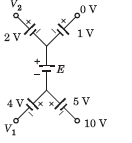Solution:

Going from 10 V to 0 V: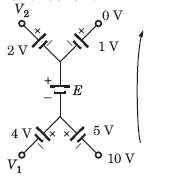• 10 + 5 + E + 1 = 0
• E = -16V
• Minus sign indicates that the polarity of battery should be reversed.
QUESTION: 8

Consider the circuit graph shown in fig. Each branch of circuit graph represent a circuit element. The value of voltage v1 is: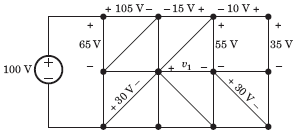Solution:
• 100 = 65 + v2
⇒ v2 = 35 V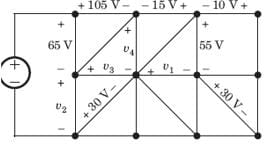• v3 - 30 = v2
⇒ v3 = 65 V
• 105 + v4 - v- 65 = 0
⇒ v4 = 25 V
• v4 + 15 - 55 + v1 = 0
⇒ v1 = 15 V
QUESTION: 9

What quantity of charge must be delivered by a battery with a Potential Difference of 110 V to do 660J of Work?

Solution:

► V = W / Q
► Q = W / V = 660 / 110 = 6 C

QUESTION: 10

Find the value of R1.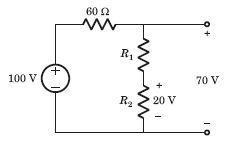Solution:
• Voltage across 60 Ω resistor = 30 V
• Current = 30 / 60 = 0.5 A
• Voltage across R1 is = (70 - 20) V = 50 V
• R1 = 50 / 0.5 = 100 Ω
QUESTION: 11

Twelve 6 Ω resistor are used as edge to form a cube.The resistance between two diagonally opposite corner of the cube is

Solution:

The current i will be distributed in the cube branches symmetrically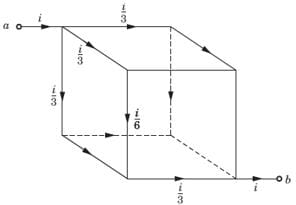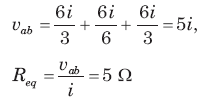QUESTION: 12

Find the value of v1.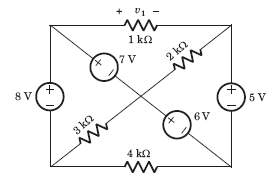Solution:

If we go from +ve side of 1 kΩ through 7 V, 6 V and 5 V
We get, v1 = 7 + 6 - 5 = 8 V

QUESTION: 13

The voltage vo in fig is always equal to: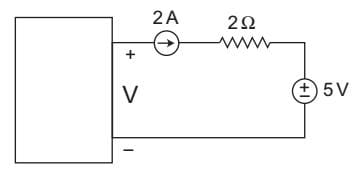Solution:

From above figure
V – 4 – 5 = 0
or
V = 9 V
Hence alternative (D) is the correct choice.

QUESTION: 14

In a AC circuit, resistive and total impedance are 10 and 20 ohms respectively. What is the phase angle difference between the voltage and current?

Solution:
• Cosɸ = R/Z; where R= 10 ohms and Z = 20 ohms
• Cosɸ = 1/2 means phase angle is 60 degree.
QUESTION: 15

The quantity of a charge that will be transferred by a current flow of 10 A over 1 hour period is _________ ?

Solution:

We know that,
I=Q / t or Q = I x t = 10 x 1 hours
{since unit of current is Cs-1, therefore time should be in seconds}
∴ Q = 10 x 60 x 60
= 36000 C
= 3.6 x 10C

QUESTION: 16

A capacitor is charged by a constant current of 2 mA and results in a voltage increase of 12 V in a 10 sec interval. The value of capacitance is:

Solution: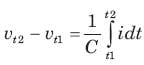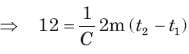12 * C  = 2 m x 10
C = 1.67 mF
Hence, Capacitance is equal to 1.67 mF.

QUESTION: 17

The energy required to charge a 10 μF capacitor to 100 V is:

Solution: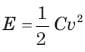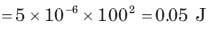QUESTION: 18

The current in a 100 μF capacitor is shown in fig. If capacitor is initially uncharged, then the waveform for the voltage across it is: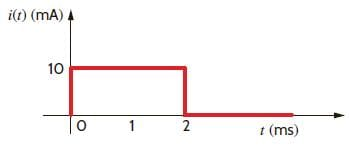Solution: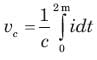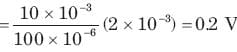This 0.2 V increases linearly from 0 to 0.2 V. Then current is zero. So capacitor hold this voltage.

QUESTION: 19

The voltage across a 100 μF capacitor is shown in fig. The waveform for the current in the capacitor is: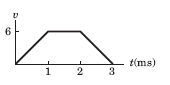Solution: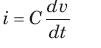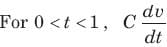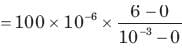= 600 mA
For 1 ms < t < 2 ms,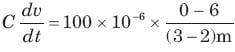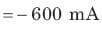QUESTION: 20

The waveform for the current in a 200 μF capacitor is shown in fig. The waveform for the capacitor voltage is: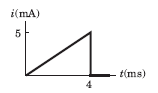Solution: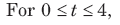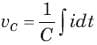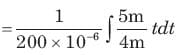= 3125 t2
At t = 4 ms, vc = 0.05 V
It will be parabolic path at t = 0
t-axis will be tangent.Use Code STAYHOME200 and get INR 200 additional OFF Use Coupon Code